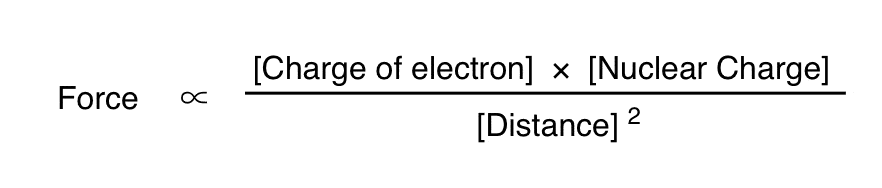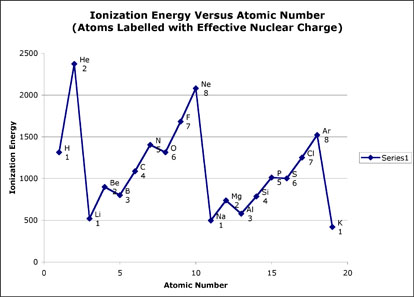# From Gen Chem to Organic Chem, Pt. 3 – Effective Nuclear Charge

Last updated: February 24th, 2020 |

If you’ve taken a physics course, you’ve probably covered the thrilling (to some) topic of electrostatics. There’s a basic formula which allows you to calculate the force between two point charges. We call this relationship Coulomb’s Law.For our purposes we’re not really interested in quantifying the magnitude of the forces. This is the point where you can make fun of organic chemists for not liking math. I prefer to think of it as that we’re more interested in understanding how different variables are related to each other. So let’s boil it down a little bit and then go into detail.We’ll call the first charge q1 the electron charge. This is always going to be –1. The second component q2 is the nuclear charge. This will vary with the number of protons in the nucleus, as we shall see. Finally, we have r, the distance between them. Finally, we’ll get rid of the equals sign and the constant to highlight the proportional nature of these relationships.One thing to note – the signs are opposite, so we’ll get a negative number, which implies an attractive force. If the charges were the same, the force would be the same but act in the opposite direction (i.e. repulsion). Secondly, while it’s true that the electrons are also going to repel each other, it’s relatively small for our purposes and we’re going to ignore it.

Two observations:

1) the force falls off in proportion to the square of the distance. So in our example, as the electron moves twice as far away from the nucleus, the force will be 1/4 as great. Remember that electrons can’t just go anywhere – their positions are determined by the orbitals. So as the orbitals fill up, the interaction between the valence electrons (the highest energy electrons) and the nucleus is going to be less.

2) Since the electronic charge is constant, the magnitude of the interaction is going to be extremely dependent on the nuclear charge.  How do we calculate the nuclear charge? Let’s look at sodium. The valence electron in sodium is in the 3s orbital. The atomic number of sodium is 11, meaning it has 11 protons in the nucleus. So naïvely we might assign +11 as the nuclear charge. This doesn’t make much sense though. If you look at the chart of ionization energies versus atomic number, they have a periodic relationship, so something more complicated is going on.What’s actually going on is that the lower energy electrons in the filled shells are shielding the nuclear charge. So to get a better picture of the true force acting on the electron, we have to account for that. We call this the effective nuclear charge, or Zeff.

If we naïvely ignore the repulsive effects of the electrons on each other [and also ignore the howls of outrage from the theoretical chemists as we do so], we can come up with a rough value for the effective nuclear charge as follows:

Zeff = atomic number – atomic number of the preceding noble gas.

Using this, we get Zeff(Na) = 11 – 10 [Ne] = 1

Using this formula, all the alkali metals have a Zeff of +1, all the alkaline earths have a Zeff of +2, the halogens are +7 and so on.

It is this number, the Zeff, which has the largest impact on the personalities of each of the classes of atoms.

So based on our equation we can confidently make several important predictions

1. Ionization energy (the energy required to take one electron away) is going to decrease as we go down the periodic table, since we are increasing the distance. This is most vividly demonstrated by the reactions of the different alkali metals with water.
2. Ionization energy is going to increase as we move across the periodic table, since we’re increasing Zeff.
3. Atomic radius will increase as we go down the periodic table, since the electrons will be less tightly held.
4. Zeff reaches a maximum at the noble gas configuration (Zeff = 8). With this electronic configuration, each electron feels the pull of 8 protons on it.

This is the deep reason for the octet rule. If we tried to add an extra electron to neon (which would have to go into the 3s orbital) the electron would feel a Zeff of 0; we’d be putting it in an orbital where the attraction between the nucleus and the electron would be shielded by the intermediate electrons. Conversely, trying to remove an electron from neon is difficult becuase each electron feels the pull of eight protons on it, which is a very powerful electrostatic interaction.

This equation also goes a long way toward explaining the electronegativity of elements, Why is fluorine the most electronegative element? Because the electrons feel the highest effective nuclear charge (Z=7) and they are the closest to the nucleus (i.e. d is the smallest).

Electronegativity has a huge effect in organic chemistry, as we will see. So understanding how it is generated from the formula above will help to give you an intuitive feel for how it works.

## Comment section

### 15 thoughts on “From Gen Chem to Organic Chem, Pt. 3 – Effective Nuclear Charge”

1.Iz says:

I’m a little confused about how effective nuclear charge relates to electronegativity and ionization energy. Effective nuclear charge is basically the charge of the nucleus “felt” by the valence electrons after the blocking effect of the shielding electrons has been taken into account, right? I don’t know why I’m confused. Maybe you can sum up ENC and how it relates to other periodic trends in other terms? I think it may be because it has been put in my mind to think of things in terms of the octet rule, rather than understanding where the octet rule itself originates and going from there. Thanks, love this blog.

1.james says:

What we commonly refer to as “electronegativity” is in fact the attractive force exerted by the nucleus towards electrons in the valence shell. With fluorine essentially you’ve got 7 protons in the nucleus [not counting the 2 whose effects are cancelled by the electrons in the 1s valence shell] exerting an attractive force on 7 electrons in hybrid orbitals of identical energy. You can imagine the effect would be less for oxygen (6 protons) and greater for neon (8 protons), a fact borne out by their measured ionization energies.

2.Anu says:

My textbook says,
Zeff = Z – S ; where,
Z is the number of protons in the nucleus (atomic number), and
S is the average number of electrons between the nucleus and the electron in question (the number of nonvalence electrons).

1.SaO. p says:

Where Z= atomic number
and S= number of non-valence electrons
For Na:
Z=11; S=10(since the valence electron, which is the electron found in the outermost shell, is 1)
Zeff=Z-S
Zeff=11-10= +1.

2.Clinton says:

You are both correct! Think back to electron configurations; there was a short hand and long hand way of writing them. The short hand allows us to ignore all the non-valence electrons and replace them instead with the preceding noble gas. The atomic number of the preceding noble gas = the number of non-valence electrons for an atom of interest. So when James says to subtract the atomic number of the preceding noble gas, that means the same thing as the equation Zeff = Z – S, because S represents the non-valence electrons.

3.Saloni says:

Hey
I’m a bit confused as well. Can you put up a diagram of what is happening here?
Thanks. :)

4.Saloni says:
5.Jounayd says:

We created this octet rule checker page in order to help the user to see if the element confirm the octet rule or not. http://mon-ip.awardspace.com/octetrule/

6.jounayd says:

You can visit my octet rule checker tool here http://mon-ip.awardspace.com/octetrule/ you have to enter the element symbol and you get the explanation

7.SaO. p says:

Thanks a whole lot for this website! My fear of gen-chem & orgo is diminishing by every read of your subsequent articles.

8.Shannon says:

You’re an angel for taking the time to explain all of this. I’ve always wondered what the mathematical/conceptual explanation for all of those numbers is. (I suppose because most chemistry classes gloss over the magnetic quantum number). This makes so much sense, thank you.

1.James Ashenhurst says:

Glad you found it helpful Shannon. The teacher I had in 3rd year quantum chem (Axel Becke) did a fantastic job of explaining all the components of the wave equation.

9.Vikram Nathan says:

This is incredibly helpful when reviewing for an upcoming Organic Chemistry course. Thank you!!

1.James Ashenhurst says: